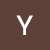# Linear Regression with added constraints on coefficients

Linear regression is used to obtain the linear relationship between dependent and independent variables. For example, we want to find the relation between individuals age and weight, where age is independent variable and weight is dependent variable.

The simple linear regression is of the form

Y=a + b*X

Where X is independent variable and Y is dependent. Slope of this line is b and y-intercept is a. Here our task is to find the coefficients a and b which minimize the error between our predicted data and actual data. Error is

Error= Actual — predicted

In the above situation there…